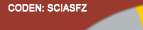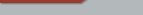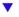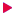| Home  | About ScienceAsia  | Publication charge  | Advertise with us  | Subscription for printed version  | Contact usEditorial Board Journal Policy Instructions for Authors Online submission Author Login Reviewer Login Volume 48 Number 3 Volume 48 Number 2 Volume 48S Number 1 Volume 48 Number 1 Volume 47 Number 6 Volume 47 Number 5 Earlier issuesVolume 43 Number 4Volume 43 Number 5 Volume 43 Number 6previous article next articleResearch articles

ScienceAsia 43 (2017): 326-333 |doi: 10.2306/scienceasia1513-1874.2017.43.326

# γ-total dominating graphs of paths and cycles

## Alongkot Wongsriyaa, Nantapath Trakultraiprukb,*

ABSTRACT:     A total dominating set for a graph G=(V(G),E(G)) is a subset D of V(G) such that every vertex in V(G) is adjacent to some vertex in D. The total domination number of G, denoted by γt(G), is the minimum cardinality of a total dominating set of G. A total dominating set of cardinality γt(G) is called a γ-total dominating set. Let TDγ be the set of all γ-total dominating sets in G. We define the γ-total dominating graph of G, denoted by TDγ(G), to be the graph whose vertex set is TDγ, and two γ-total dominating sets D1 and D2 from TDγ are adjacent in TDγ(G) if D1=D2∖{u}∪{v} for some uD2 and vD2. In this paper, we present γ-total dominating graphs of paths and cycles.